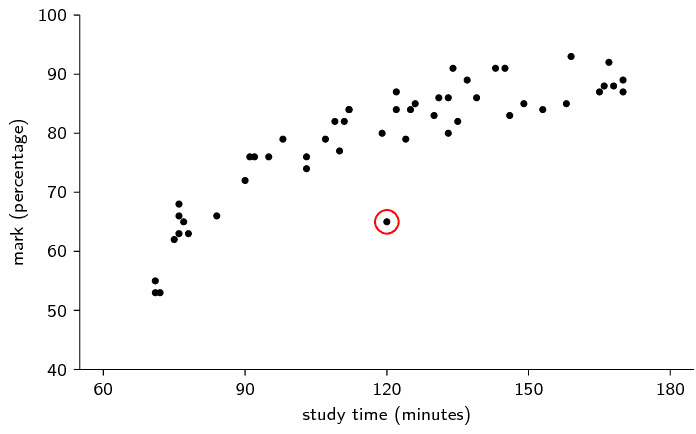Home Practice
For learners and parents For teachers and schools
Textbooks
Full catalogue
Pricing SupportLog in

We think you are located in United States. Is this correct?

# 11.6 Identification of outliers

## 11.6 Identification of outliers (EMBKH)

temp text

An outlier in a data set is a value that is far away from the rest of the values in the data set. In a box and whisker diagram, outliers are usually close to the whiskers of the diagram. This is because the centre of the diagram represents the data between the first and third quartiles, which is where $$\text{50}\%$$ of the data lie, while the whiskers represent the extremes — the minimum and maximum — of the data.

## Worked example 12: Identifying outliers

Find the outliers in the following data set by drawing a box and whisker diagram and locating the data values on the diagram.

$$\text{0,5}$$ ; $$\text{1}$$ ; $$\text{1,1}$$ ; $$\text{1,4}$$ ; $$\text{2,4}$$ ; $$\text{2,8}$$ ; $$\text{3,5}$$ ; $$\text{5,1}$$ ; $$\text{5,2}$$ ; $$\text{6}$$ ; $$\text{6,5}$$ ; $$\text{9,5}$$

### Determine the five number summary

The minimum of the data set is $$\text{0,5}$$. The maximum of the data set is $$\text{9,5}$$. Since there are $$\text{12}$$ values in the data set, the median lies between the sixth and seventh values, making it equal to $$\frac{\text{2,8}+\text{3,5}}{2} = \text{3,15}$$. The first quartile lies between the third and fourth values, making it equal to $$\frac{\text{1,1}+\text{1,4}}{2} = \text{1,25}$$. The third quartile lies between the ninth and tenth values, making it equal to $$\frac{\text{5,2}+\text{6}}{2} = \text{5,6}$$.

### Draw the box and whisker diagram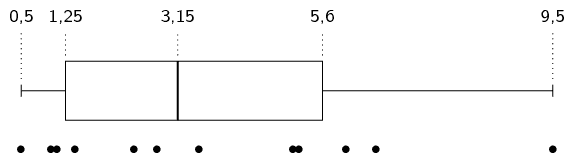In the figure above, each value in the data set is shown with a black dot.

### Find the outliers

From the diagram we can see that most of the values are between $$\text{1}$$ and $$\text{6}$$. The only value that is very far away from this range is the maximum at $$\text{9,5}$$. Therefore $$\text{9,5}$$ is the only outlier in the data set.

You should also be able to identify outliers in plots of two variables. A scatter plot is a graph that shows the relationship between two random variables. We call these data bivariate (literally meaning two variables) and we plot the data for two different variables on one set of axes. The following example shows what a typical scatter plot looks like. For Grade $$\text{11}$$ you do not need to learn how to draw these $$\text{2}$$-dimensional scatter plots, but you should be able to identify outliers on them. As before, an outlier is a value that is far removed from the main distribution of data.

## Worked example 13: Scatter plot

We have a data set that relates the heights and weights of a number of people. The height is the first variable and its value is plotted along the horizontal axis. The weight is the second variable and its value is plotted along the vertical axis. The data values are shown on the plot below. Identify any outliers on the scatter plot.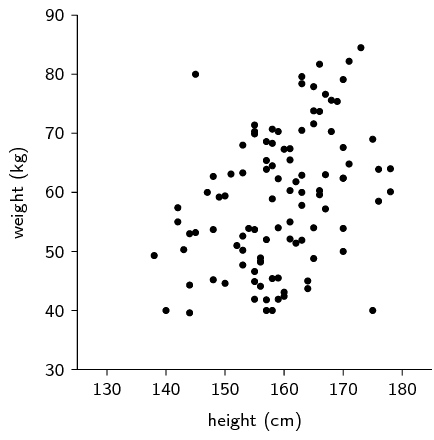We inspect the plot visually and notice that there are two points that lie far away from the main data distribution. These two points are circled in the plot below.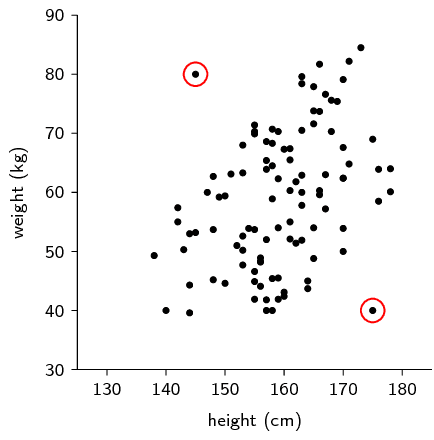## Outliers

Textbook Exercise 11.6

For each of the following data sets, draw a box and whisker diagram and determine whether there are any outliers in the data.

$$\text{30}$$ ; $$\text{21,4}$$ ; $$\text{39,4}$$ ; $$\text{33,4}$$ ; $$\text{21,1}$$ ; $$\text{29,3}$$ ; $$\text{32,8}$$ ; $$\text{31,6}$$ ; $$\text{36}$$ ;

$$\text{27,9}$$ ; $$\text{27,3}$$ ; $$\text{29,4}$$ ; $$\text{29,1}$$ ; $$\text{38,6}$$ ; $$\text{33,8}$$ ; $$\text{29,1}$$ ; $$\text{37,1}$$

Below is the box-and-whisker diagram of the data as well as dots representing the data themselves. Note that learners do not neeed to draw the dots, but this helps us to see that there are two outliers on the left.$$\text{198}$$ ; $$\text{166}$$ ; $$\text{175}$$ ; $$\text{147}$$ ; $$\text{125}$$ ; $$\text{194}$$ ; $$\text{119}$$ ; $$\text{170}$$ ; $$\text{142}$$ ; $$\text{148}$$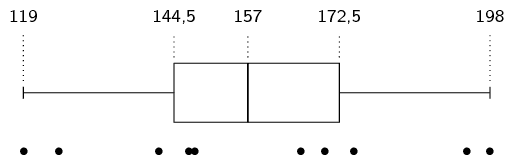There are no outliers.

$$\text{7,1}$$ ; $$\text{9,6}$$ ; $$\text{6,3}$$ ; $$-\text{5,9}$$ ; $$\text{0,7}$$ ; $$-\text{0,1}$$ ; $$\text{4,4}$$ ; $$-\text{11,7}$$ ; $$\text{10}$$ ; $$\text{2,3}$$ ; $$-\text{3,7}$$ ; $$\text{5,8}$$ ; $$-\text{1,4}$$ ; $$\text{1,7}$$ ; $$-\text{0,7}$$There is one outlier on the left.

A class's results for a test were recorded along with the amount of time spent studying for it. The results are given below. Identify any outliers in the data.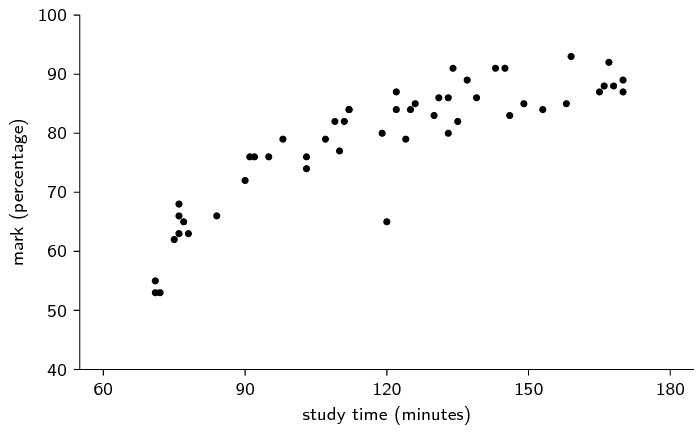There is one outlier, marked in red below.